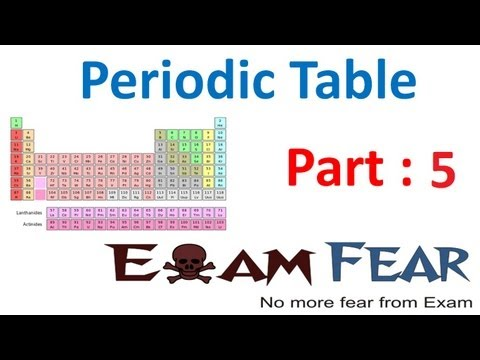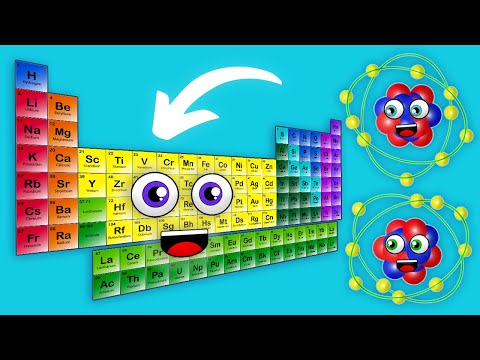Courses

Test: Mendeleev's Periodic Table

10 Questions MCQ Test Chemistry for JEE | Test: Mendeleev's Periodic Table

Description
Attempt Test: Mendeleev's Periodic Table | 10 questions in 10 minutes | Mock test for JEE preparation | Free important questions MCQ to study Chemistry for JEE for JEE Exam | Download free PDF with solutions
QUESTION: 1

The rows of the periodic table are called:

Solution:

The rows of the periodic table are called periods, while the columns of the table are called groups.

QUESTION: 2

Who was the scientist credited with devising the first periodic table similar to the one we use today?​

Solution:

The Russian scientist Dmitri Mendeleev proposed a periodic table similar to the one we use today in 1869. He arranged the elements according to "periodic law", where element properties could be predicted based on recurring similarities between elements (periodicity).

QUESTION: 3

The primary difference between the modern periodic table and Mendeleev's periodic table is:

Solution:

The modern periodic table orders elements by increasing atomic number, which is the number of protons in the atom of the element. Mendeleev did not know about the parts of the atom, so he used the next best thing -- atomic weight.

QUESTION: 4

As you move from left to right across the periodic table:

Solution:

Even though each atom has more electrons as you move from left to right across the periodic table, the atomic radius decreases. The reason is that you're also adding more protons, which exert a stronger attractive force on the electrons, drawing them in a tiny bit closer. Ionic radius also decreases, although not for the exact same reason.

QUESTION: 5

As you move from top to bottom down the periodic table:

Solution:

As you move down a periodic table group, electronegativity decreases because the distance between the atomic nucleus and the valence electrons increases.

QUESTION: 6

Which of the following are all properties of nonmetals?

Solution:

There are several ways to tell metals and nonmetals apart. Nonmetalsdon't have a metallic appearance. Unlike metals, they typically have lower melting and boiling points and tend not to conduct heat or electricity very well.

QUESTION: 7

Which of the following element groups are considered types of metals?

Solution:

About 75% of the elements on the periodic table are metals. The only groups that are not metals are the noble gases, halogens, and the group actually called nonmetals.

QUESTION: 8

Where would you find the smallest atoms of elements in the periodic table?

Solution:

Helium is the smallest atom. The energy level of the electrons determine the atom's volume. Electrons have a negative charge and the nucleus is positive since it is made up of protons and neutrons. Helium and Hydrogen have one and two electrons. This means they are in the the first energy sub level which is 1S (It can hold up to two electrons) So if that's all we were to consider, both atoms would be the same size. However, since Helium has more protons and electrons, the electrons have a greater attraction to the nucleus, thus decreasing its volume. So it is wrong to just look at atomic number. Hydrogen is not the smallest atom, it is the second smallest and Helium is the smallest.

QUESTION: 9

Electron affinity reflects the ability of an atom to accept an electron. Which is true of the alkaline earths?

Solution:

In order for an atom to have a high electron affinity, it needs to be in a position to accept electrons. The alkaline earth metals (like calcium and magnesium) have filled s subshells, so they are stable. If anything, the alkaline earths prefer to lose electrons and exist as cations.

QUESTION: 10

With respect to electron affinity, which statement applies to the halogens?

Solution:

Halogens have maximum negative electron gain enthalpy in the corresponding periods. This is due to the fact that the atoms of these elements have only one electron less than stable noble gas configurations. Electrons gain enthalpy of the elements of the group becomes less negative down the group. However, the negative electron gain enthalpy of fluorine.Use Code STAYHOME200 and get INR 200 additional OFF Use Coupon Code

Track your progress, build streaks, highlight & save important lessons and more!

Similar ContentRelated tests